Engineering Entrance Sample Papers

BITSAT Maths Sample Paper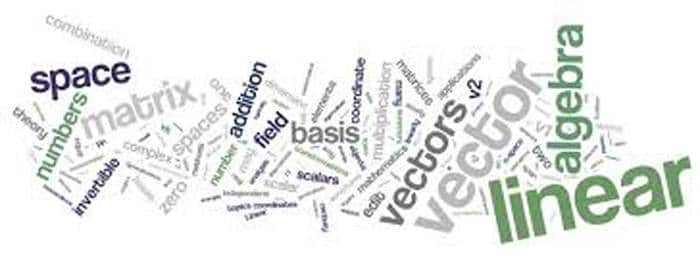BITSAT Maths Sample Question paper 1 consist 45 questions with answers based on 11th and 12th Mathematics syllabus of CBSE Board. You can download PDF of this BITSAT Math sample paper by using the link below 10th question.

Related: BITSAT Exam Pattern

Question:

If the point (1, 4) lies inside the circle x2 + y2– 6x – 10y + p = 0 and the circle neither touches nor intersects the coordinate axes, then
(a) 0 < p < 39
(b) 25 < p < 29
(c) 9 < p < 25
(d) 9 < p < 29
Ans: (b)

Question:

A student is allowed to select utmost n books from a collection of (2n + 1) books. If the total number of ways in which he can select one book is 63, then the value of n is
(a) 2
(b) 3
(c) 4
(d) None of these
Ans: (b)

Question:

If (1 + x)n = C0 + C1x + C2x2 + … + Cxxx, then the value of C0 + 2C1 + 3C2 + … + (n + 1)Cn will be
(a) (n + 2)2n – 1
(b) (n + 1)2nj
(c) (n + 1)2n – 1
(d) (n + 2)2n
Ans: (a)

Question:

Equation of chord (AB) of circle x2 + y2 = 2 passing through the point P (2, 2), such that PB/PA = 3, is
(a) x = 3y
(b) x = y
(c) y  = (x – 2)
(d) none of these
Ans: (b)

Question:

The mean and the variance of a binomial distribution are 4 and 2 respectively. Then the probability of 2 successes is
(a) 28/256
(b) 219/256
(c) 128/256
(d) 37/256
Ans: (a)

Question:

A point P moves in such a way that the ratio of its distances from two coplanar points is always fixed number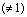(≠1). Then its locus is
(a) Straight line
(b) Circle
(c) Parabola
(d) A pair of straight lines
Ans: (b)

Question:

An unbiased die with faces marked 1, 2, 3, 4, 5 and 6 is rolled four times. Out of four face values obtained the probability that the minimum face value is not less than 2 and the maximum  face value is not greater than 5, is
(a) 16/81
(b) 1/81
(c) 80/81
(d) 65/81
Ans: (a)

Question:

Volume of tetrahedron formed by the planes x + y = 0, y + z = 0, z + x = 0, x + y + z – 1 = 0 is
(a) 1/6
(b) 1/3
(c) 2/3
(d) none of these
Ans: (c)

Question:

In a room there are 12 bulbs of the same voltage, each having a separate switch. The number of ways to light the room with different amount of illumination is
(a) 122 – 1
(b) 212
(c) 212 – 1
(d) none of these
Ans: (c)

Question:

The ends of the latus rectum of the conic x2 + 10x – 16y + 25 = 0 are
(a) (3, – 4), (13, 4)
(b) (- 3, – 4), (13, – 4)
(c) (3, 4), (- 13, 4)
(d) (5, – 8), (- 5, 8)
Ans: (c)

Question:

Two cards are drawn from a well shuffled pack of cards with replacement. The probability of drawing both aces is
(a) (1/13)2
(b) 1/13 + 1/17
(c) 1/12 x 1/51
(d) 1/13 x 4/51
Ans: (a)

Question:

Let Sn denote the sum to n terms of an arithmetic progression whose first term is a. If the common difference is equal to Sn – kSn – 1 + Sn – 2, then k =
(a) 1
(b) 2
(c) 3
(d) none of these
Ans: (b)

Question:

If ax2 + bx + c = 0 and bx2 + cx + a = 0, a ¹ 0, b ¹ 0 have a common root, then the value of a3 + b3 + c3 – 3abc is
(a) 0
(b) 1
(c) 2
(d) 10
Ans: (a)

Question:

The motion of a particle along a straight line is described by the function x = (2t – 3)2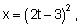, where x is in metre and t is in second. Then the velocity of the particle at origin is :
(a) 0
(b) 1
(c) 2
(d) none of these
Ans: (a)

Question:

If m is the root of the equation (1 – ab)x2 – (a2 + b2)x – (1 + ab) = 0, and m harmonic means are inserted between a and b, then the difference between the last and the first of the means equals
(a) ab(a – b)
(b) a(b – a)
(c) ab(b – a)
(d) b – a
Ans: (c)

Related: UPSEE Maths Sample Papers

Question:

If z =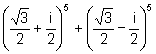, then

(a) Re(z) = 0
(b) Im z = 0
(c) Re(z) > 0, Im(z) > 0
(d) Re(z) > 0
Ans: (b)

Question:

The solution of differential equation (ex + 1)ydy = (y + 1)exdx is :
(a) (ex + 1) (y + 1) = cey
(b) (ex + 1) | (y + 1) | = ce–y
(c) (ex + 1) (y + 1) = ±cey
(d) none of these
Ans: (a)

Question:

Find the integral factor of equation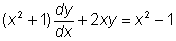(a)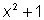(b)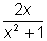(c)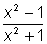(d) None of these
Ans: (a)

Question:

In any triangle AB = 2, BC = 4, CA = 3 and D is mid-point of BC, then
(a) cos B = 11/6
(b) cos B = 7/8
Ans: (d)

Question:

Equation of ellipse with foci (5, 0) and (-5, 0) and 5x – 36 = 0 as one directrix, is
(a) 11x+ 36y= 196
(b) 11x+ 18y= 396
(c) 11x+ 18y= 198
(d) 11x+ 36y= 396
Ans: (d)

Related: BITSAT Chemistry Questions

Question:

The angle between the pair of straight lines represented by 2x2 – 7xy + 3y2 = 0 is
(a) 60o
(b) 45o
(c) tan–1 (7/6)
(d) 30o
Ans: (b)

Question:

Mean of 100 items is 49. It was discovered that 3 items 60, 70, 80 were wrongly read as 38, 22, 50 respectively. The correct mean is
(a) 48
(b) 78
(c) 50
(d) 80